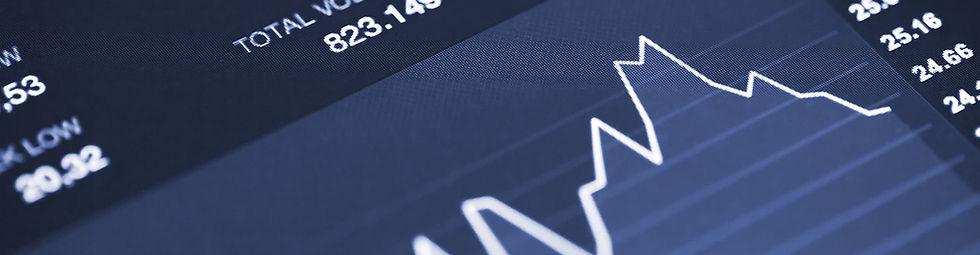### Aggregate Expenditure

#### Aggregate Expenditure Model

###### What is the Aggregate Expenditure Model?

The aggregate expenditure model is a model that measures the effect of an increase in one or more components of aggregate expenditure on real Gross Domestic Product.

###### What does the Aggregate Expenditure Model Look Like?###### Elements of the Aggregate Expenditure Model

Expenditure (Y Axis)

This is used to show the effect of an initial change in one of more components of aggregate expenditure. E meaning the level of expenditure.

Real GDP (X Axis)

This is used to show the multiplier effect and the greater change in RGDP from an initial change in Expenditure.

45º Line

The line that shows where real GDP equals expenditure.